moveit2 The MoveIt Motion Planning Framework for ROS 2.
moveit::core::RobotModel Class Reference

Definition of a kinematic model. This class is not thread safe, however multiple instances can be created. More...

`#include <robot_model.h>`

Collaboration diagram for moveit::core::RobotModel: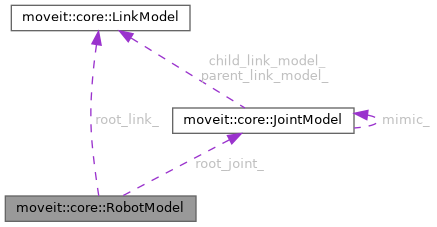[legend]

## Public Member Functions

RobotModel (const urdf::ModelInterfaceSharedPtr &urdf_model, const srdf::ModelConstSharedPtr &srdf_model)
Construct a kinematic model from a parsed description and a list of planning groups. More...

~RobotModel ()
Destructor. Clear all memory. More...

const std::string & getName () const
Get the model name. More...

const std::string & getModelFrame () const
Get the frame in which the transforms for this model are computed (when using a RobotState). This frame depends on the root joint. As such, the frame is either extracted from SRDF, or it is assumed to be the name of the root link. More...

bool isEmpty () const
Return true if the model is empty (has no root link, no joints) More...

const urdf::ModelInterfaceSharedPtr & getURDF () const
Get the parsed URDF model. More...

const srdf::ModelConstSharedPtr & getSRDF () const
Get the parsed SRDF model. More...

void printModelInfo (std::ostream &out) const
Print information about the constructed model. More...

void getVariableRandomPositions (random_numbers::RandomNumberGenerator &rng, double *values) const
Compute the random values for a RobotState. More...

void getVariableDefaultPositions (double *values) const
Compute the default values for a RobotState. More...

void getVariableRandomPositions (random_numbers::RandomNumberGenerator &rng, std::vector< double > &values) const
Compute the random values for a RobotState. More...

void getVariableDefaultPositions (std::vector< double > &values) const
Compute the default values for a RobotState. More...

void getVariableRandomPositions (random_numbers::RandomNumberGenerator &rng, std::map< std::string, double > &values) const
Compute the random values for a RobotState. More...

void getVariableDefaultPositions (std::map< std::string, double > &values) const
Compute the default values for a RobotState. More...

bool enforcePositionBounds (double *state) const

bool enforcePositionBounds (double *state, const JointBoundsVector &active_joint_bounds) const

bool satisfiesPositionBounds (const double *state, double margin=0.0) const

bool satisfiesPositionBounds (const double *state, const JointBoundsVector &active_joint_bounds, double margin=0.0) const

double getMaximumExtent () const

double getMaximumExtent (const JointBoundsVector &active_joint_bounds) const

double distance (const double *state1, const double *state2) const
Return the sum of joint distances between two states. An L1 norm. Only considers active joints. More...

void interpolate (const double *from, const double *to, double t, double *state) const

std::size_t getVariableCount () const
Get the number of variables that describe this model. More...

const std::vector< std::string > & getVariableNames () const
Get the names of the variables that make up the joints that form this state. Fixed joints have no DOF, so they are not here, but the variables for mimic joints are included. The number of returned elements is always equal to getVariableCount() More...

const VariableBoundsgetVariableBounds (const std::string &variable) const
Get the bounds for a specific variable. Throw an exception of variable is not found. More...

const JointBoundsVectorgetActiveJointModelsBounds () const
Get the bounds for all the active joints. More...

void getMissingVariableNames (const std::vector< std::string > &variables, std::vector< std::string > &missing_variables) const

size_t getVariableIndex (const std::string &variable) const
Get the index of a variable in the robot state. More...

const JointModelgetCommonRoot (const JointModel *a, const JointModel *b) const
Get the deepest joint in the kinematic tree that is a common parent of both joints passed as argument. More...

void setKinematicsAllocators (const std::map< std::string, SolverAllocatorFn > &allocators)
A map of known kinematics solvers (associated to their group name) More...

const JointModelgetRootJoint () const
Get the root joint. There will be one root joint unless the model is empty. This is either extracted from the SRDF, or a fixed joint is assumed, if no specification is given. More...

const std::string & getRootJointName () const
Return the name of the root joint. Throws an exception if there is no root joint. More...

bool hasJointModel (const std::string &name) const
Check if a joint exists. Return true if it does. More...

const JointModelgetJointModel (const std::string &joint) const
Get a joint by its name. Output error and return nullptr when the joint is missing. More...

const JointModelgetJointModel (size_t index) const
Get a joint by its index. Output error and return nullptr when the link is missing. More...

JointModelgetJointModel (const std::string &joint)
Get a joint by its name. Output error and return nullptr when the joint is missing. More...

const std::vector< const JointModel * > & getJointModels () const
Get the array of joints, in the order they appear in the robot state. More...

const std::vector< JointModel * > & getJointModels ()
Get the array of joints, in the order they appear in the robot state. This includes all types of joints (including mimic & fixed), as opposed to JointModelGroup::getJointModels(). More...

const std::vector< std::string > & getJointModelNames () const
Get the array of joint names, in the order they appear in the robot state. More...

const std::vector< const JointModel * > & getActiveJointModels () const
Get the array of joints that are active (not fixed, not mimic) in this model. More...

const std::vector< std::string > & getActiveJointModelNames () const
Get the array of active joint names, in the order they appear in the robot state. More...

const std::vector< JointModel * > & getActiveJointModels ()
Get the array of joints that are active (not fixed, not mimic) in this model. More...

const std::vector< const JointModel * > & getSingleDOFJointModels () const
This is a list of all single-dof joints (including mimic joints) More...

const std::vector< const JointModel * > & getMultiDOFJointModels () const
This is a list of all multi-dof joints. More...

const std::vector< const JointModel * > & getContinuousJointModels () const
Get the array of continuous joints, in the order they appear in the robot state. More...

const std::vector< const JointModel * > & getMimicJointModels () const
Get the array of mimic joints, in the order they appear in the robot state. More...

const JointModelgetJointOfVariable (int variable_index) const

const JointModelgetJointOfVariable (const std::string &variable) const

std::size_t getJointModelCount () const

bool hasJointModelGroup (const std::string &group) const
Check if the JointModelGroup group exists. More...

const JointModelGroupgetJointModelGroup (const std::string &name) const
Get a joint group from this model (by name) More...

JointModelGroupgetJointModelGroup (const std::string &name)
Get a joint group from this model (by name) More...

const std::vector< const JointModelGroup * > & getJointModelGroups () const
Get the available joint groups. More...

const std::vector< JointModelGroup * > & getJointModelGroups ()
Get the available joint groups. More...

const std::vector< std::string > & getJointModelGroupNames () const
Get the names of all groups that are defined for this model. More...

bool hasEndEffector (const std::string &eef) const
Check if an end effector exists. More...

const JointModelGroupgetEndEffector (const std::string &name) const
Get the joint group that corresponds to a given end-effector name. More...

JointModelGroupgetEndEffector (const std::string &name)
Get the joint group that corresponds to a given end-effector name. More...

const std::vector< const JointModelGroup * > & getEndEffectors () const
Get the map between end effector names and the groups they correspond to. More...

## Protected Member Functions

Get the transforms between link and all its rigidly attached descendants. More...

const JointModelcomputeCommonRoot (const JointModel *a, const JointModel *b) const
Given two joints, find their common root. More...

void updateMimicJoints (double *values) const
Update the variable values for the state of a group with respect to the mimic joints. More...

## Protected Attributes

std::string model_name_
The name of the robot. More...

std::string model_frame_
The reference (base) frame for this model. The frame is either extracted from the SRDF as a virtual joint, or it is assumed to be the name of the root link in the URDF. More...

srdf::ModelConstSharedPtr srdf_

urdf::ModelInterfaceSharedPtr urdf_

The first physical link for the robot. More...

A map from link names to their instances. More...

The vector of links that are updated when computeTransforms() is called, in the order they are updated. More...

The vector of links that are updated when computeTransforms() is called, in the order they are updated. More...

Only links that have collision geometry specified. More...

Total number of geometric shapes in this model. More...

const JointModelroot_joint_
The root joint. More...

JointModelMap joint_model_map_
A map from joint names to their instances. More...

std::vector< JointModel * > joint_model_vector_
The vector of joints in the model, in the order they appear in the state vector. More...

std::vector< const JointModel * > joint_model_vector_const_
The vector of joints in the model, in the order they appear in the state vector. More...

std::vector< std::string > joint_model_names_vector_
The vector of joint names that corresponds to joint_model_vector_. More...

std::vector< JointModel * > active_joint_model_vector_
The vector of joints in the model, in the order they appear in the state vector. More...

std::vector< std::string > active_joint_model_names_vector_
The vector of joint names that corresponds to active_joint_model_vector_. More...

std::vector< const JointModel * > active_joint_model_vector_const_
The vector of joints in the model, in the order they appear in the state vector. More...

std::vector< const JointModel * > continuous_joint_model_vector_
The set of continuous joints this model contains. More...

std::vector< const JointModel * > mimic_joints_
The set of mimic joints this model contains. More...

std::vector< const JointModel * > single_dof_joints_

std::vector< const JointModel * > multi_dof_joints_

std::vector< int > common_joint_roots_
For every two joints, the index of the common root for the joints is stored. More...

std::vector< std::string > variable_names_
The names of the DOF that make up this state (this is just a sequence of joint variable names; not necessarily joint names!) More...

std::size_t variable_count_
Get the number of variables necessary to describe this model. More...

VariableIndexMap joint_variables_index_map_
The state includes all the joint variables that make up the joints the state consists of. This map gives the position in the state vector of the group for each of these variables. Additionally, it includes the names of the joints and the index for the first variable of that joint. More...

std::vector< int > active_joint_model_start_index_

JointBoundsVector active_joint_models_bounds_
The bounds for all the active joint models. More...

std::vector< const JointModel * > joints_of_variable_
The joints that correspond to each variable index. More...

JointModelGroupMap joint_model_group_map_
A map from group names to joint groups. More...

JointModelGroupMap end_effectors_map_
The known end effectors. More...

std::vector< JointModelGroup * > joint_model_groups_
The array of joint model groups, in alphabetical order. More...

std::vector< const JointModelGroup * > joint_model_groups_const_
The array of joint model groups, in alphabetical order. More...

std::vector< std::string > joint_model_group_names_
A vector of all group names, in alphabetical order. More...

std::vector< const JointModelGroup * > end_effectors_
The array of end-effectors, in alphabetical order. More...

Get the physical root link of the robot. More...

const std::string & getRootLinkName () const
Get the name of the root link of the robot. More...

bool hasLinkModel (const std::string &name) const
Check if a link exists. Return true if it does. More...

Get a link by its name. Output error and return nullptr when the link is missing. More...

Get a link by its index. Output error and return nullptr when the link is missing. More...

Get a link by its name. Output error and return nullptr when the link is missing. More...

More...

More...

const std::vector< std::string > & getLinkModelNames () const

Get the link models that have some collision geometry associated to themselves. More...

const std::vector< std::string > & getLinkModelNamesWithCollisionGeometry () const
Get the names of the link models that have some collision geometry associated to themselves. More...

Get the latest link upwards the kinematic tree, which is only connected via fixed joints. More...

## Detailed Description

Definition of a kinematic model. This class is not thread safe, however multiple instances can be created.

Definition at line 75 of file robot_model.h.

## ◆ RobotModel()

 moveit::core::RobotModel::RobotModel ( const urdf::ModelInterfaceSharedPtr & urdf_model, const srdf::ModelConstSharedPtr & srdf_model )

Construct a kinematic model from a parsed description and a list of planning groups.

Definition at line 54 of file robot_model.cpp.

## ◆ ~RobotModel()

 moveit::core::RobotModel::~RobotModel ( )

Destructor. Clear all memory.

Definition at line 62 of file robot_model.cpp.

## ◆ computeCommonRoot()

 const JointModel* moveit::core::RobotModel::computeCommonRoot ( const JointModel * a, const JointModel * b ) const
protected

Given two joints, find their common root.

## ◆ computeFixedTransforms()

protected

Get the transforms between link and all its rigidly attached descendants.

Definition at line 1630 of file robot_model.cpp.

Here is the call graph for this function: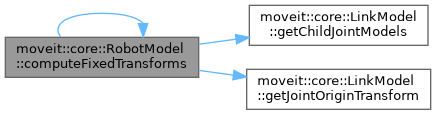## ◆ distance()

 double moveit::core::RobotModel::distance ( const double * state1, const double * state2 ) const

Return the sum of joint distances between two states. An L1 norm. Only considers active joints.

Definition at line 1481 of file robot_model.cpp.

## ◆ enforcePositionBounds() [1/2]

 bool moveit::core::RobotModel::enforcePositionBounds ( double * state ) const
inline

Definition at line 339 of file robot_model.h.

Here is the caller graph for this function: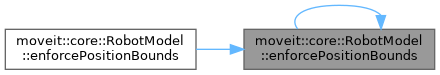## ◆ enforcePositionBounds() [2/2]

 bool moveit::core::RobotModel::enforcePositionBounds ( double * state, const JointBoundsVector & active_joint_bounds ) const

Definition at line 1466 of file robot_model.cpp.

Here is the call graph for this function: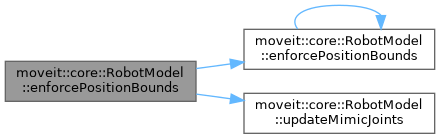## ◆ getActiveJointModelNames()

 const std::vector& moveit::core::RobotModel::getActiveJointModelNames ( ) const
inline

Get the array of active joint names, in the order they appear in the robot state.

Definition at line 176 of file robot_model.h.

## ◆ getActiveJointModels() [1/2]

 const std::vector& moveit::core::RobotModel::getActiveJointModels ( )
inline

Get the array of joints that are active (not fixed, not mimic) in this model.

Definition at line 182 of file robot_model.h.

## ◆ getActiveJointModels() [2/2]

 const std::vector& moveit::core::RobotModel::getActiveJointModels ( ) const
inline

Get the array of joints that are active (not fixed, not mimic) in this model.

Definition at line 170 of file robot_model.h.

## ◆ getActiveJointModelsBounds()

 const JointBoundsVector& moveit::core::RobotModel::getActiveJointModelsBounds ( ) const
inline

Get the bounds for all the active joints.

Definition at line 439 of file robot_model.h.

## ◆ getCommonRoot()

 const JointModel* moveit::core::RobotModel::getCommonRoot ( const JointModel * a, const JointModel * b ) const
inline

Get the deepest joint in the kinematic tree that is a common parent of both joints passed as argument.

Definition at line 451 of file robot_model.h.

Here is the call graph for this function:Here is the caller graph for this function:## ◆ getContinuousJointModels()

 const std::vector& moveit::core::RobotModel::getContinuousJointModels ( ) const
inline

Get the array of continuous joints, in the order they appear in the robot state.

Definition at line 201 of file robot_model.h.

## ◆ getEndEffector() [1/2]

 JointModelGroup * moveit::core::RobotModel::getEndEffector ( const std::string & name )

Get the joint group that corresponds to a given end-effector name.

Definition at line 486 of file robot_model.cpp.

## ◆ getEndEffector() [2/2]

 const JointModelGroup * moveit::core::RobotModel::getEndEffector ( const std::string & name ) const

Get the joint group that corresponds to a given end-effector name.

Definition at line 472 of file robot_model.cpp.

Here is the caller graph for this function: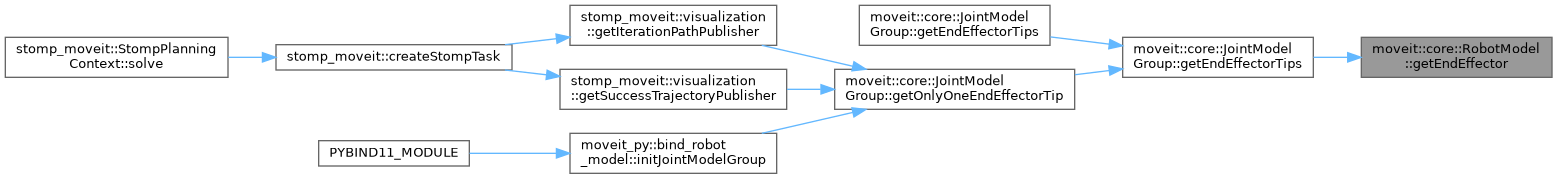## ◆ getEndEffectors()

 const std::vector& moveit::core::RobotModel::getEndEffectors ( ) const
inline

Get the map between end effector names and the groups they correspond to.

Definition at line 410 of file robot_model.h.

Here is the caller graph for this function:## ◆ getJointModel() [1/3]

 JointModel * moveit::core::RobotModel::getJointModel ( const std::string & joint )

Get a joint by its name. Output error and return nullptr when the joint is missing.

Definition at line 1316 of file robot_model.cpp.

## ◆ getJointModel() [2/3]

 const JointModel * moveit::core::RobotModel::getJointModel ( const std::string & joint ) const

Get a joint by its name. Output error and return nullptr when the joint is missing.

Definition at line 1296 of file robot_model.cpp.

## ◆ getJointModel() [3/3]

 const JointModel * moveit::core::RobotModel::getJointModel ( size_t index ) const

Get a joint by its index. Output error and return nullptr when the link is missing.

Definition at line 1305 of file robot_model.cpp.

## ◆ getJointModelCount()

 std::size_t moveit::core::RobotModel::getJointModelCount ( ) const
inline

Definition at line 223 of file robot_model.h.

## ◆ getJointModelGroup() [1/2]

 JointModelGroup * moveit::core::RobotModel::getJointModelGroup ( const std::string & name )

Get a joint group from this model (by name)

Definition at line 516 of file robot_model.cpp.

## ◆ getJointModelGroup() [2/2]

 const JointModelGroup * moveit::core::RobotModel::getJointModelGroup ( const std::string & name ) const

Get a joint group from this model (by name)

Definition at line 505 of file robot_model.cpp.

Here is the caller graph for this function: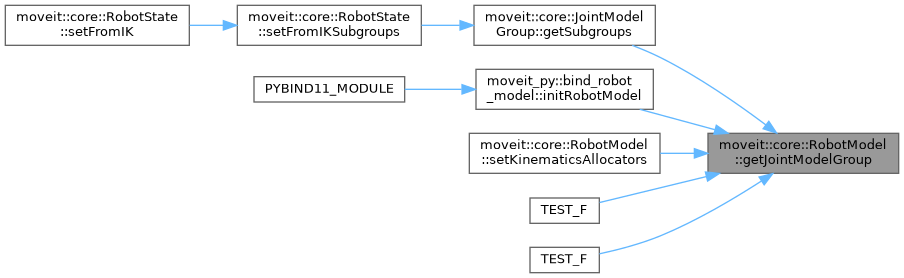## ◆ getJointModelGroupNames()

 const std::vector& moveit::core::RobotModel::getJointModelGroupNames ( ) const
inline

Get the names of all groups that are defined for this model.

Definition at line 395 of file robot_model.h.

Here is the caller graph for this function:## ◆ getJointModelGroups() [1/2]

 const std::vector& moveit::core::RobotModel::getJointModelGroups ( )
inline

Get the available joint groups.

Definition at line 389 of file robot_model.h.

## ◆ getJointModelGroups() [2/2]

 const std::vector& moveit::core::RobotModel::getJointModelGroups ( ) const
inline

Get the available joint groups.

Definition at line 383 of file robot_model.h.

Here is the caller graph for this function:## ◆ getJointModelNames()

 const std::vector& moveit::core::RobotModel::getJointModelNames ( ) const
inline

Get the array of joint names, in the order they appear in the robot state.

Definition at line 164 of file robot_model.h.

## ◆ getJointModels() [1/2]

 const std::vector& moveit::core::RobotModel::getJointModels ( )
inline

Get the array of joints, in the order they appear in the robot state. This includes all types of joints (including mimic & fixed), as opposed to JointModelGroup::getJointModels().

Definition at line 158 of file robot_model.h.

## ◆ getJointModels() [2/2]

 const std::vector& moveit::core::RobotModel::getJointModels ( ) const
inline

Get the array of joints, in the order they appear in the robot state.

Definition at line 150 of file robot_model.h.

## ◆ getJointOfVariable() [1/2]

 const JointModel* moveit::core::RobotModel::getJointOfVariable ( const std::string & variable ) const
inline

Definition at line 218 of file robot_model.h.

Here is the call graph for this function:## ◆ getJointOfVariable() [2/2]

 const JointModel* moveit::core::RobotModel::getJointOfVariable ( int variable_index ) const
inline

Definition at line 213 of file robot_model.h.

Here is the caller graph for this function:inline

Definition at line 305 of file robot_model.h.

 LinkModel * moveit::core::RobotModel::getLinkModel ( const std::string & link, bool * has_link = `nullptr` )

Get a link by its name. Output error and return nullptr when the link is missing.

Definition at line 1341 of file robot_model.cpp.

 const LinkModel * moveit::core::RobotModel::getLinkModel ( const std::string & link, bool * has_link = `nullptr` ) const

Get a link by its name. Output error and return nullptr when the link is missing.

Definition at line 1325 of file robot_model.cpp.

Here is the caller graph for this function: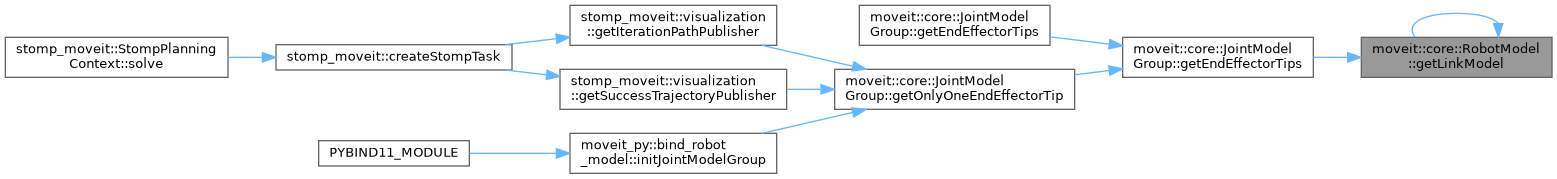Get a link by its index. Output error and return nullptr when the link is missing.

Definition at line 1330 of file robot_model.cpp.

inline

Definition at line 300 of file robot_model.h.

 const std::vector& moveit::core::RobotModel::getLinkModelNames ( ) const
inline

Definition at line 283 of file robot_model.h.

 const std::vector& moveit::core::RobotModel::getLinkModelNamesWithCollisionGeometry ( ) const
inline

Get the names of the link models that have some collision geometry associated to themselves.

Definition at line 295 of file robot_model.h.

inline

Definition at line 277 of file robot_model.h.

 const std::vector& moveit::core::RobotModel::getLinkModels ( ) const
inline

Definition at line 271 of file robot_model.h.

 const std::vector& moveit::core::RobotModel::getLinkModelsWithCollisionGeometry ( ) const
inline

Get the link models that have some collision geometry associated to themselves.

Definition at line 289 of file robot_model.h.

## ◆ getMaximumExtent() [1/2]

 double moveit::core::RobotModel::getMaximumExtent ( ) const
inline

Definition at line 350 of file robot_model.h.

## ◆ getMaximumExtent() [2/2]

 double moveit::core::RobotModel::getMaximumExtent ( const JointBoundsVector & active_joint_bounds ) const

Definition at line 1442 of file robot_model.cpp.

## ◆ getMimicJointModels()

 const std::vector& moveit::core::RobotModel::getMimicJointModels ( ) const
inline

Get the array of mimic joints, in the order they appear in the robot state.

Definition at line 208 of file robot_model.h.

## ◆ getMissingVariableNames()

 void moveit::core::RobotModel::getMissingVariableNames ( const std::vector< std::string > & variables, std::vector< std::string > & missing_variables ) const

Definition at line 1419 of file robot_model.cpp.

Here is the call graph for this function: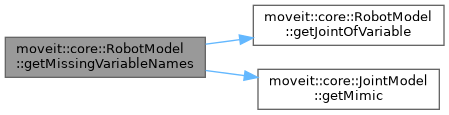## ◆ getModelFrame()

 const std::string& moveit::core::RobotModel::getModelFrame ( ) const
inline

Get the frame in which the transforms for this model are computed (when using a RobotState). This frame depends on the root joint. As such, the frame is either extracted from SRDF, or it is assumed to be the name of the root link.

Definition at line 94 of file robot_model.h.

Here is the caller graph for this function:## ◆ getMultiDOFJointModels()

 const std::vector& moveit::core::RobotModel::getMultiDOFJointModels ( ) const
inline

This is a list of all multi-dof joints.

Definition at line 194 of file robot_model.h.

## ◆ getName()

 const std::string& moveit::core::RobotModel::getName ( ) const
inline

Get the model name.

Definition at line 85 of file robot_model.h.

Here is the caller graph for this function:static

Get the latest link upwards the kinematic tree, which is only connected via fixed joints.

Definition at line 1360 of file robot_model.cpp.

Here is the call graph for this function:## ◆ getRootJoint()

 const JointModel * moveit::core::RobotModel::getRootJoint ( ) const

Get the root joint. There will be one root joint unless the model is empty. This is either extracted from the SRDF, or a fixed joint is assumed, if no specification is given.

Definition at line 72 of file robot_model.cpp.

Here is the caller graph for this function: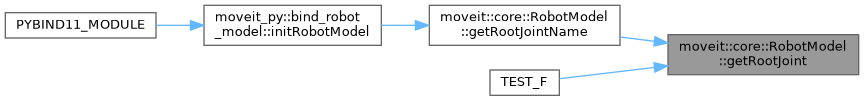## ◆ getRootJointName()

 const std::string& moveit::core::RobotModel::getRootJointName ( ) const
inline

Return the name of the root joint. Throws an exception if there is no root joint.

Definition at line 131 of file robot_model.h.

Here is the call graph for this function:Here is the caller graph for this function:Get the physical root link of the robot.

Definition at line 77 of file robot_model.cpp.

Here is the caller graph for this function: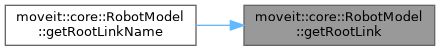const std::string& moveit::core::RobotModel::getRootLinkName ( ) const
inline

Get the name of the root link of the robot.

Definition at line 238 of file robot_model.h.

Here is the call graph for this function:## ◆ getSingleDOFJointModels()

 const std::vector& moveit::core::RobotModel::getSingleDOFJointModels ( ) const
inline

This is a list of all single-dof joints (including mimic joints)

Definition at line 188 of file robot_model.h.

## ◆ getSRDF()

 const srdf::ModelConstSharedPtr& moveit::core::RobotModel::getSRDF ( ) const
inline

Get the parsed SRDF model.

Definition at line 112 of file robot_model.h.

## ◆ getURDF()

 const urdf::ModelInterfaceSharedPtr& moveit::core::RobotModel::getURDF ( ) const
inline

Get the parsed URDF model.

Definition at line 106 of file robot_model.h.

Here is the caller graph for this function:## ◆ getVariableBounds()

 const VariableBounds& moveit::core::RobotModel::getVariableBounds ( const std::string & variable ) const
inline

Get the bounds for a specific variable. Throw an exception of variable is not found.

Definition at line 433 of file robot_model.h.

Here is the call graph for this function:Here is the caller graph for this function:## ◆ getVariableCount()

 std::size_t moveit::core::RobotModel::getVariableCount ( ) const
inline

Get the number of variables that describe this model.

Definition at line 418 of file robot_model.h.

Here is the caller graph for this function: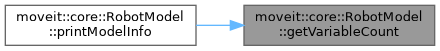## ◆ getVariableDefaultPositions() [1/3]

 void moveit::core::RobotModel::getVariableDefaultPositions ( double * values ) const

Compute the default values for a RobotState.

Definition at line 1403 of file robot_model.cpp.

Here is the call graph for this function: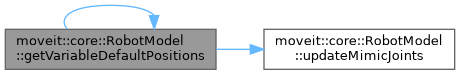Here is the caller graph for this function: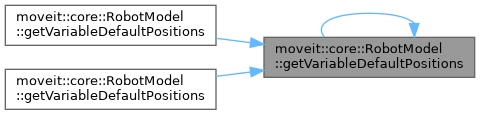## ◆ getVariableDefaultPositions() [2/3]

 void moveit::core::RobotModel::getVariableDefaultPositions ( std::map< std::string, double > & values ) const

Compute the default values for a RobotState.

Definition at line 1410 of file robot_model.cpp.

Here is the call graph for this function: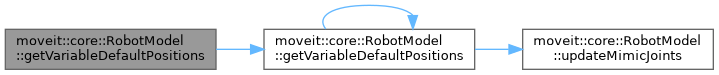## ◆ getVariableDefaultPositions() [3/3]

 void moveit::core::RobotModel::getVariableDefaultPositions ( std::vector< double > & values ) const
inline

Compute the default values for a RobotState.

Definition at line 326 of file robot_model.h.

Here is the call graph for this function:## ◆ getVariableIndex()

 size_t moveit::core::RobotModel::getVariableIndex ( const std::string & variable ) const

Get the index of a variable in the robot state.

Definition at line 1434 of file robot_model.cpp.

Here is the caller graph for this function: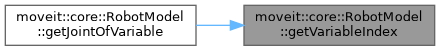## ◆ getVariableNames()

 const std::vector& moveit::core::RobotModel::getVariableNames ( ) const
inline

Get the names of the variables that make up the joints that form this state. Fixed joints have no DOF, so they are not here, but the variables for mimic joints are included. The number of returned elements is always equal to getVariableCount()

Definition at line 427 of file robot_model.h.

## ◆ getVariableRandomPositions() [1/3]

 void moveit::core::RobotModel::getVariableRandomPositions ( random_numbers::RandomNumberGenerator & rng, double * values ) const

Compute the random values for a RobotState.

Definition at line 1386 of file robot_model.cpp.

Here is the call graph for this function: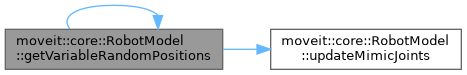Here is the caller graph for this function: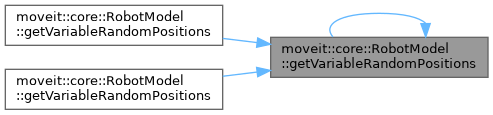## ◆ getVariableRandomPositions() [2/3]

 void moveit::core::RobotModel::getVariableRandomPositions ( random_numbers::RandomNumberGenerator & rng, std::map< std::string, double > & values ) const

Compute the random values for a RobotState.

Definition at line 1393 of file robot_model.cpp.

Here is the call graph for this function: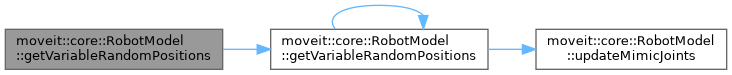## ◆ getVariableRandomPositions() [3/3]

 void moveit::core::RobotModel::getVariableRandomPositions ( random_numbers::RandomNumberGenerator & rng, std::vector< double > & values ) const
inline

Compute the random values for a RobotState.

Definition at line 319 of file robot_model.h.

Here is the call graph for this function:## ◆ hasEndEffector()

 bool moveit::core::RobotModel::hasEndEffector ( const std::string & eef ) const

Check if an end effector exists.

Definition at line 467 of file robot_model.cpp.

## ◆ hasJointModel()

 bool moveit::core::RobotModel::hasJointModel ( const std::string & name ) const

Check if a joint exists. Return true if it does.

Definition at line 1286 of file robot_model.cpp.

## ◆ hasJointModelGroup()

 bool moveit::core::RobotModel::hasJointModelGroup ( const std::string & group ) const

Check if the JointModelGroup group exists.

Definition at line 500 of file robot_model.cpp.

Here is the caller graph for this function: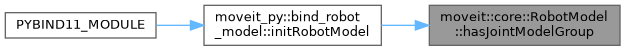bool moveit::core::RobotModel::hasLinkModel ( const std::string & name ) const

Check if a link exists. Return true if it does.

If this is followed by a call to getLinkModel(), better use the latter with the has_link argument

Definition at line 1291 of file robot_model.cpp.

Here is the caller graph for this function: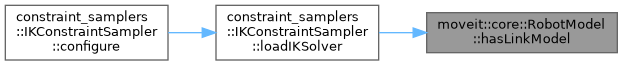## ◆ interpolate()

 void moveit::core::RobotModel::interpolate ( const double * from, const double * to, double t, double * state ) const

Interpolate between "from" state, to "to" state. Mimic joints are correctly updated.

Parameters
 from interpolate from this state to to this state t a fraction in the range [0 1]. If 1, the result matches "to" state exactly. state holds the result

Definition at line 1493 of file robot_model.cpp.

Here is the call graph for this function: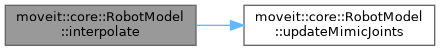## ◆ isEmpty()

 bool moveit::core::RobotModel::isEmpty ( ) const
inline

Return true if the model is empty (has no root link, no joints)

Definition at line 100 of file robot_model.h.

## ◆ printModelInfo()

 void moveit::core::RobotModel::printModelInfo ( std::ostream & out ) const

Print information about the constructed model.

Definition at line 1579 of file robot_model.cpp.

Here is the call graph for this function: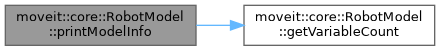## ◆ satisfiesPositionBounds() [1/2]

 bool moveit::core::RobotModel::satisfiesPositionBounds ( const double * state, const JointBoundsVector & active_joint_bounds, double margin = `0.0` ) const

Definition at line 1453 of file robot_model.cpp.

Here is the call graph for this function: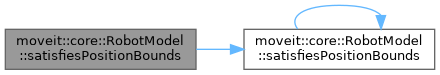## ◆ satisfiesPositionBounds() [2/2]

 bool moveit::core::RobotModel::satisfiesPositionBounds ( const double * state, double margin = `0.0` ) const
inline

Definition at line 344 of file robot_model.h.

Here is the caller graph for this function: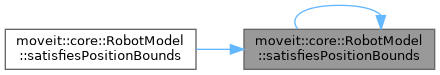## ◆ setKinematicsAllocators()

 void moveit::core::RobotModel::setKinematicsAllocators ( const std::map< std::string, SolverAllocatorFn > & allocators )

A map of known kinematics solvers (associated to their group name)

Definition at line 1507 of file robot_model.cpp.

Here is the call graph for this function: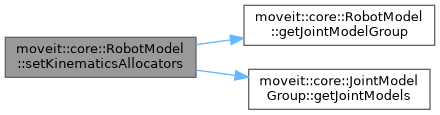## ◆ updateMimicJoints()

 void moveit::core::RobotModel::updateMimicJoints ( double * values ) const
protected

Update the variable values for the state of a group with respect to the mimic joints.

Definition at line 1376 of file robot_model.cpp.

Here is the caller graph for this function: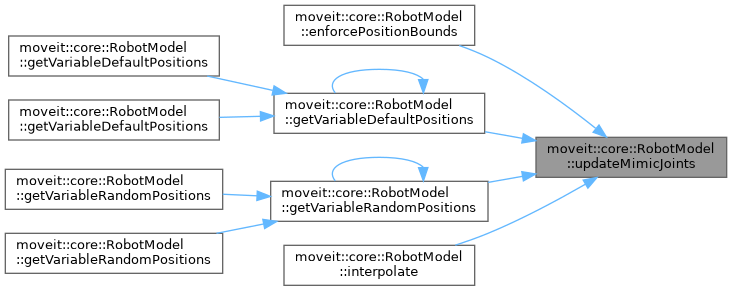## ◆ active_joint_model_names_vector_

 std::vector moveit::core::RobotModel::active_joint_model_names_vector_
protected

The vector of joint names that corresponds to active_joint_model_vector_.

Definition at line 534 of file robot_model.h.

## ◆ active_joint_model_start_index_

 std::vector moveit::core::RobotModel::active_joint_model_start_index_
protected

Definition at line 572 of file robot_model.h.

## ◆ active_joint_model_vector_

 std::vector moveit::core::RobotModel::active_joint_model_vector_
protected

The vector of joints in the model, in the order they appear in the state vector.

Definition at line 531 of file robot_model.h.

## ◆ active_joint_model_vector_const_

 std::vector moveit::core::RobotModel::active_joint_model_vector_const_
protected

The vector of joints in the model, in the order they appear in the state vector.

Definition at line 537 of file robot_model.h.

## ◆ active_joint_models_bounds_

 JointBoundsVector moveit::core::RobotModel::active_joint_models_bounds_
protected

The bounds for all the active joint models.

Definition at line 575 of file robot_model.h.

## ◆ common_joint_roots_

 std::vector moveit::core::RobotModel::common_joint_roots_
protected

For every two joints, the index of the common root for the joints is stored.

for jointA, jointB the index of the common root is located in the array at location jointA->getJointIndex() * nr.joints + jointB->getJointIndex(). The size of this array is nr.joints * nr.joints

Definition at line 556 of file robot_model.h.

## ◆ continuous_joint_model_vector_

 std::vector moveit::core::RobotModel::continuous_joint_model_vector_
protected

The set of continuous joints this model contains.

Definition at line 540 of file robot_model.h.

## ◆ end_effectors_

 std::vector moveit::core::RobotModel::end_effectors_
protected

The array of end-effectors, in alphabetical order.

Definition at line 598 of file robot_model.h.

## ◆ end_effectors_map_

 JointModelGroupMap moveit::core::RobotModel::end_effectors_map_
protected

The known end effectors.

Definition at line 586 of file robot_model.h.

## ◆ joint_model_group_map_

 JointModelGroupMap moveit::core::RobotModel::joint_model_group_map_
protected

A map from group names to joint groups.

Definition at line 583 of file robot_model.h.

## ◆ joint_model_group_names_

 std::vector moveit::core::RobotModel::joint_model_group_names_
protected

A vector of all group names, in alphabetical order.

Definition at line 595 of file robot_model.h.

## ◆ joint_model_groups_

 std::vector moveit::core::RobotModel::joint_model_groups_
protected

The array of joint model groups, in alphabetical order.

Definition at line 589 of file robot_model.h.

## ◆ joint_model_groups_const_

 std::vector moveit::core::RobotModel::joint_model_groups_const_
protected

The array of joint model groups, in alphabetical order.

Definition at line 592 of file robot_model.h.

## ◆ joint_model_map_

 JointModelMap moveit::core::RobotModel::joint_model_map_
protected

A map from joint names to their instances.

Definition at line 519 of file robot_model.h.

## ◆ joint_model_names_vector_

 std::vector moveit::core::RobotModel::joint_model_names_vector_
protected

The vector of joint names that corresponds to joint_model_vector_.

Definition at line 528 of file robot_model.h.

## ◆ joint_model_vector_

 std::vector moveit::core::RobotModel::joint_model_vector_
protected

The vector of joints in the model, in the order they appear in the state vector.

Definition at line 522 of file robot_model.h.

## ◆ joint_model_vector_const_

 std::vector moveit::core::RobotModel::joint_model_vector_const_
protected

The vector of joints in the model, in the order they appear in the state vector.

Definition at line 525 of file robot_model.h.

## ◆ joint_variables_index_map_

 VariableIndexMap moveit::core::RobotModel::joint_variables_index_map_
protected

The state includes all the joint variables that make up the joints the state consists of. This map gives the position in the state vector of the group for each of these variables. Additionally, it includes the names of the joints and the index for the first variable of that joint.

Definition at line 570 of file robot_model.h.

## ◆ joints_of_variable_

 std::vector moveit::core::RobotModel::joints_of_variable_
protected

The joints that correspond to each variable index.

Definition at line 578 of file robot_model.h.

protected

Total number of geometric shapes in this model.

Definition at line 511 of file robot_model.h.

protected

A map from link names to their instances.

Definition at line 493 of file robot_model.h.

protected

Definition at line 502 of file robot_model.h.

protected

Definition at line 508 of file robot_model.h.

protected

The vector of links that are updated when computeTransforms() is called, in the order they are updated.

Definition at line 496 of file robot_model.h.

protected

The vector of links that are updated when computeTransforms() is called, in the order they are updated.

Definition at line 499 of file robot_model.h.

protected

Only links that have collision geometry specified.

Definition at line 505 of file robot_model.h.

## ◆ mimic_joints_

 std::vector moveit::core::RobotModel::mimic_joints_
protected

The set of mimic joints this model contains.

Definition at line 543 of file robot_model.h.

## ◆ model_frame_

 std::string moveit::core::RobotModel::model_frame_
protected

The reference (base) frame for this model. The frame is either extracted from the SRDF as a virtual joint, or it is assumed to be the name of the root link in the URDF.

Definition at line 481 of file robot_model.h.

## ◆ model_name_

 std::string moveit::core::RobotModel::model_name_
protected

The name of the robot.

Definition at line 477 of file robot_model.h.

## ◆ multi_dof_joints_

 std::vector moveit::core::RobotModel::multi_dof_joints_
protected

Definition at line 547 of file robot_model.h.

## ◆ root_joint_

 const JointModel* moveit::core::RobotModel::root_joint_
protected

The root joint.

Definition at line 516 of file robot_model.h.

protected

The first physical link for the robot.

Definition at line 490 of file robot_model.h.

## ◆ single_dof_joints_

 std::vector moveit::core::RobotModel::single_dof_joints_
protected

Definition at line 545 of file robot_model.h.

## ◆ srdf_

 srdf::ModelConstSharedPtr moveit::core::RobotModel::srdf_
protected

Definition at line 483 of file robot_model.h.

## ◆ urdf_

 urdf::ModelInterfaceSharedPtr moveit::core::RobotModel::urdf_
protected

Definition at line 485 of file robot_model.h.

## ◆ variable_count_

 std::size_t moveit::core::RobotModel::variable_count_
protected

Get the number of variables necessary to describe this model.

Definition at line 565 of file robot_model.h.

## ◆ variable_names_

 std::vector moveit::core::RobotModel::variable_names_
protected

The names of the DOF that make up this state (this is just a sequence of joint variable names; not necessarily joint names!)

Definition at line 562 of file robot_model.h.

The documentation for this class was generated from the following files: